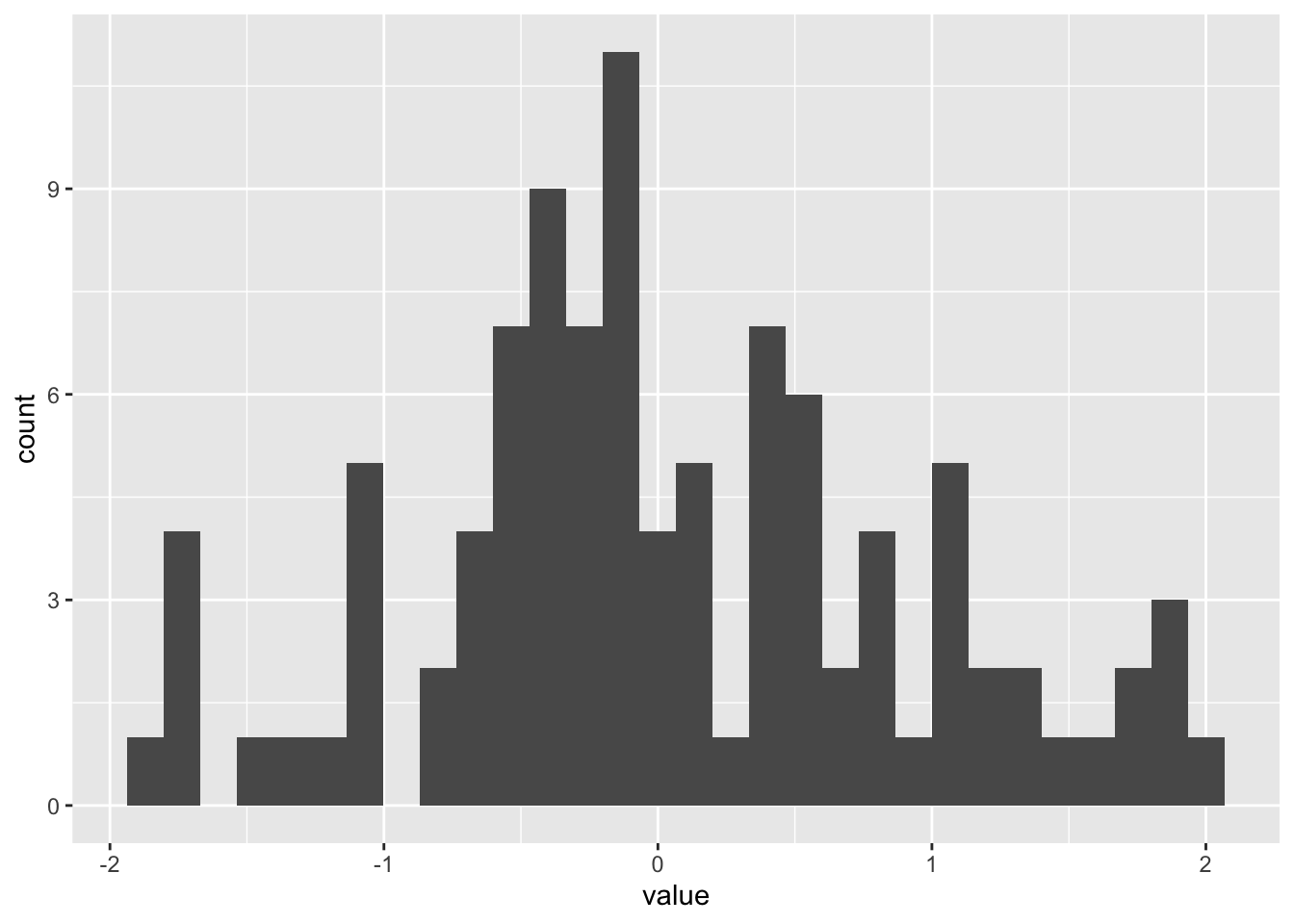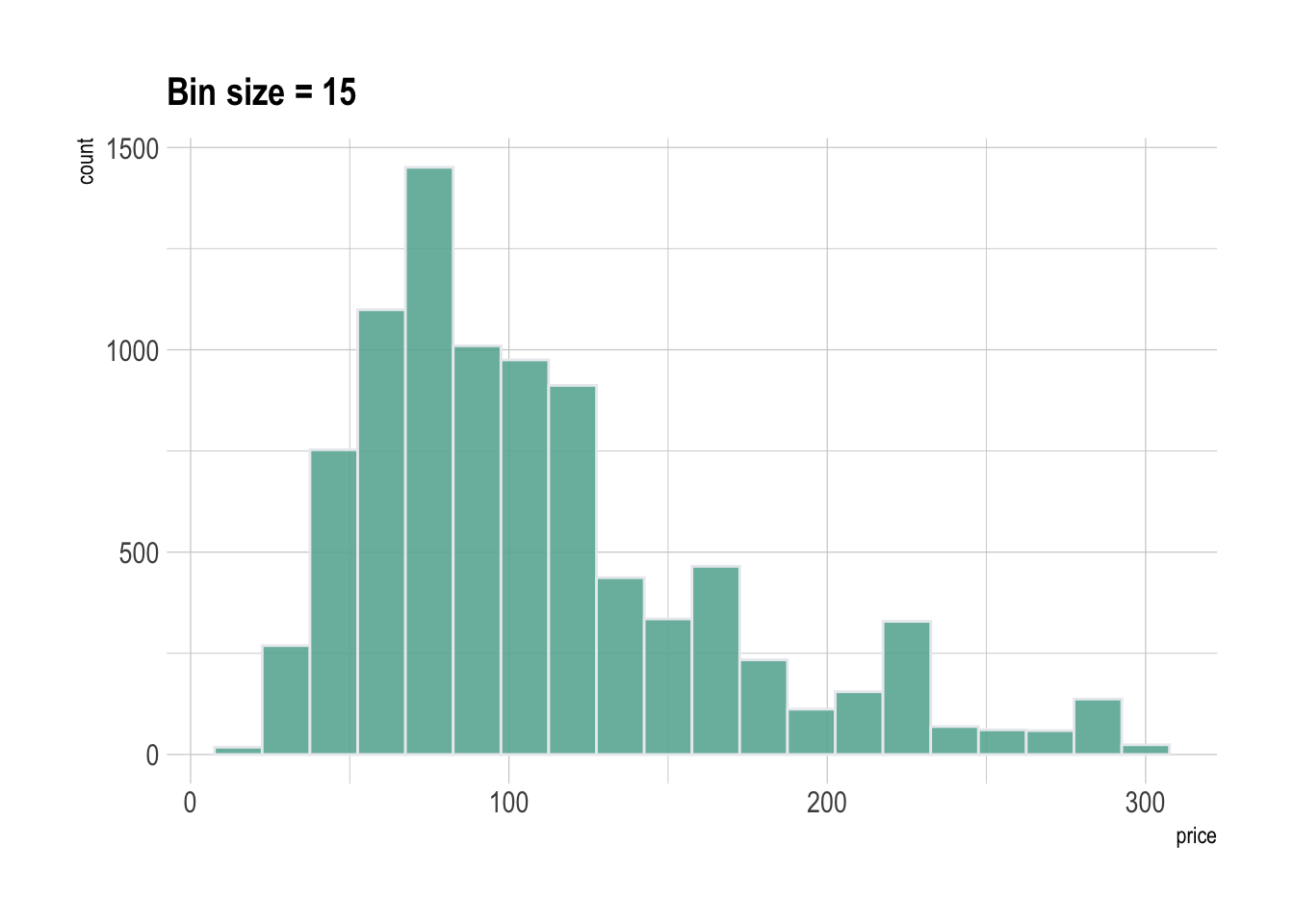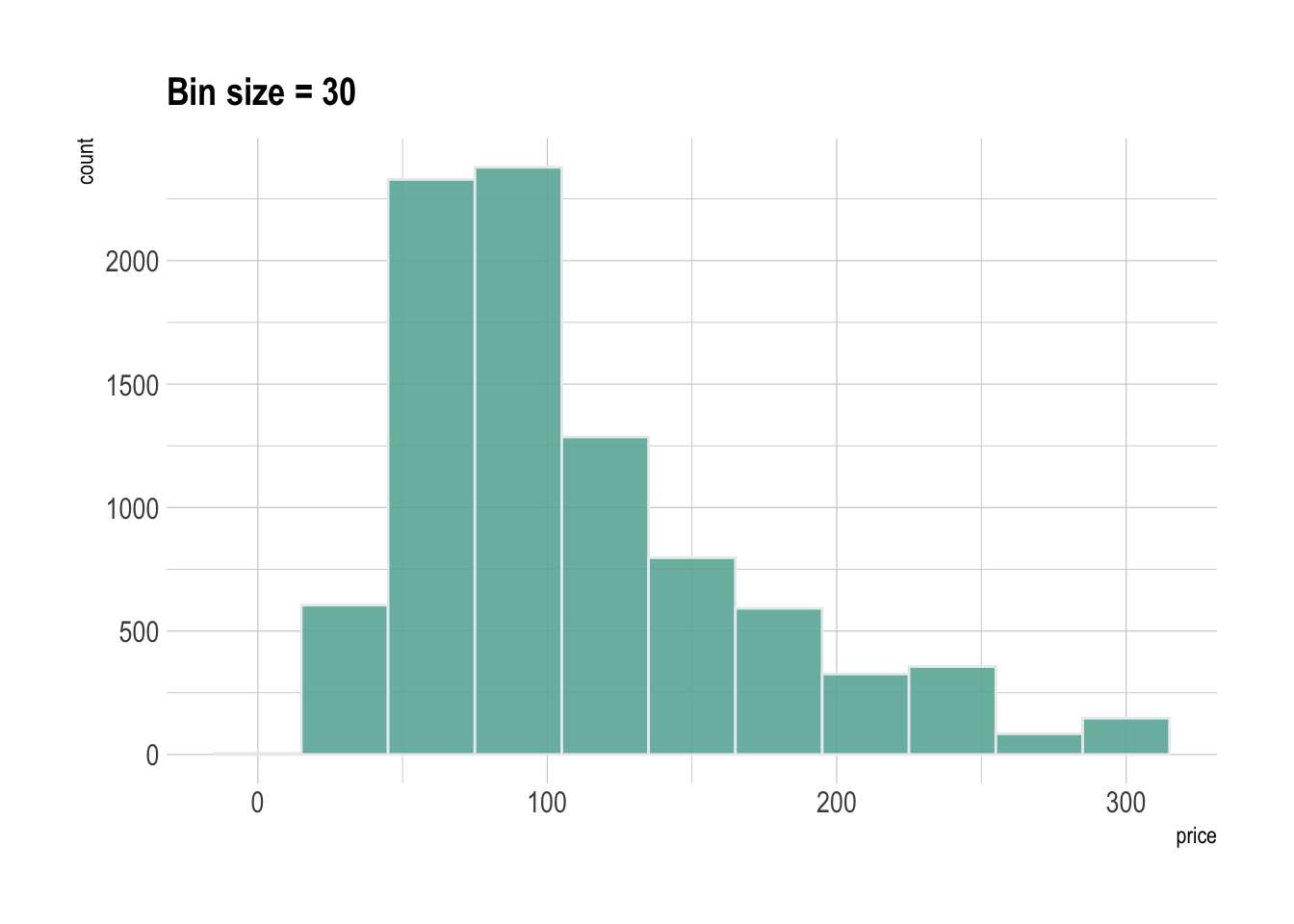# Basic histogram with ggplot2

A histogram is a representation of the distribution of a numeric variable. This document explains how to build it with R and the ggplot2 package. You can find more examples in the [histogram section](histogram.html.

# Basic histogram with `geom_histogram`

It is relatively straightforward to build a histogram with `ggplot2` thanks to the `geom_histogram()` function. Only one numeric variable is needed in the input. Note that a warning message is triggered with this code: we need to take care of the bin width as explained in the next section.

``````# library
library(ggplot2)

# dataset:
data=data.frame(value=rnorm(100))

# basic histogram
p <- ggplot(data, aes(x=value)) +
geom_histogram()

#p``````# Control bin size with `binwidth`

A histogram takes as input a numeric variable and cuts it into several bins. Playing with the bin size is a very important step, since its value can have a big impact on the histogram appearance and thus on the message you’re trying to convey. This concept is explained in depth in data-to-viz.

Ggplot2 makes it a breeze to change the bin size thanks to the `binwidth` argument of the `geom_histogram` function. See below the impact it can have on the output.

``````# Libraries
library(tidyverse)
library(hrbrthemes)

# Load dataset from github
data <- read.table("https://raw.githubusercontent.com/holtzy/data_to_viz/master/Example_dataset/1_OneNum.csv", header=TRUE)

# plot
p <- data %>%
filter( price<300 ) %>%
ggplot( aes(x=price)) +
geom_histogram( binwidth=3, fill="#69b3a2", color="#e9ecef", alpha=0.9) +
ggtitle("Bin size = 3") +
theme_ipsum() +
theme(
plot.title = element_text(size=15)
)
#p``````Related chart types

## Contact

This document is a work by Yan Holtz. Any feedback is highly encouraged. You can fill an issue on Github, drop me a message on Twitter, or send an email pasting yan.holtz.data with gmail.com.